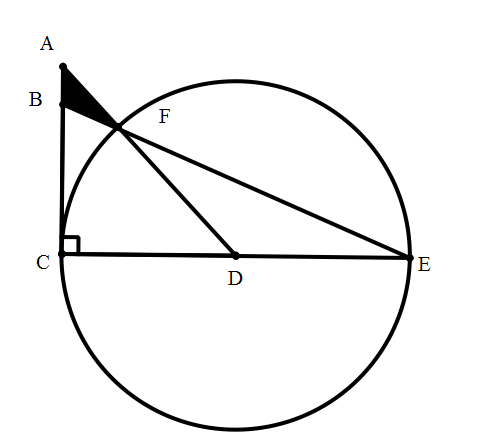# Intersecting Triangles

Geometry Level 4Diagram above shows a circle with center $D$, with $C,E,F$ be points lying on the circle, and two right triangles $ACD$ and $BCE$ with their hypotenuse intersect at $F$. If we are given $AC = CD = 10 \text{ cm}$. What is the area of triangle $ABF$ to 4 decimal places.

Details and Assumptions

• You may use the approximation: $\sqrt 2 = 1.41421356$
×

Problem Loading...

Note Loading...

Set Loading...